233
3C Tecnología. Glosas de innovación aplicadas a la pyme. ISSN: 2254 – 4143 Edición Especial Special Issue Abril 2020
COMPARATIVE ANALYSIS OF SUPERVISED MACHINE
LEARNING ALGORITHMS FOR HEART DISEASE
DETECTION
Hector Daniel Huapaya
Member of the articial intelligence research group of the faculty of systems engineering, department of
software engineering at the National University Mayor de San Marcos, Lima, (Perú).
E-mail: hector.huapaya@unmsm.edu.pe ORCID: https://orcid.org/0000-0003-3616-9046
Ciro Rodriguez
Professor at the School of Software Engineering at the National University Mayor de San Marcos, Lima,
(Perú).
E-mail: crodriguezro@unmsm.edu.pe ORCID: https://orcid.org/0000-0003-2112-1349
Doris Esenarro
Professor at the Faculty of Environmental Engineering and Graduate School of the National University
Federico Villarreal, Lima, (Perú).
E-mail: desenarro@unfv.edu.pe ORCID: https://orcid.org/0000-0002-7186-9614
Recepción:
27/12/2019
Aceptación:
30/03/2020
Publicación:
30/04/2020
Citación sugerida Suggested citation
Hector Daniel Huapaya, H. D., Rodriguez, C., y Esenarro, D. (2020). Comparative analysis of
supervised machine learning algorithms for heart disease detection. 3C Tecnología. Glosas de innovación
aplicadas a la pyme. Edición Especial, Abril 2020, 233-247. http://doi.org/10.17993/3ctecno.2020.
specialissue5.233-247234
http://doi.org/10.17993/3ctecno.2020.specialissue5.233-247
3C Tecnología. Glosas de innovación aplicadas a la pyme. ISSN: 2254 – 4143 Edición Especial Special Issue Abril 2020
ABSTRACT
This paper describes the most prominent algorithms of Supervised Machine Learning
(SML), their characteristics, and comparatives in the way of treating data. The Heart
Disease dataset obtained from Kaggle was used to determine and test its highest percentage
of accuracy. To achieve the objective, Python sklearn libraries were used to implement the
selected algorithms, evaluate and determine which algorithm is the one that obtains the best
results, applying decision tree algorithms achieved the best prediction results.
KEYWORDS
Supervised machine learning, Heart disease, Decision tree algorithms, Prediction.235
http://doi.org/10.17993/3ctecno.2020.specialissue5.233-247
3C Tecnología. Glosas de innovación aplicadas a la pyme. ISSN: 2254 – 4143 Edición Especial Special Issue Abril 2020
1. INTRODUCTION
Machine learning is one of the fastest-growing areas of computer science (Srivastava et al.,
2014), with long-range applications, which refers to the automatic detection of signicant
patterns in data with machine learning tools, which give programs the ability to learn and
Machine learning has become one of the pillars of information technology and, with that,
a reasonably central, though generally hidden, part of our life. With the increasing amount
of data available, there is a good reason to believe that intelligent data analysis will be even
more widespread as a necessary ingredient for technological progress.
There are several applications for Machine Learning (ML), being one of the most important
data mining (Bustamante, Rodríguez, & Esenarro, 2019). The handling of a large amount
of data makes people more likely to make mistakes during analyzes or, possibly, when trying
to establish relationships between multiple characteristics.
Data mining and machine learning go hand in hand with which several ideas can be
derived through appropriate learning algorithms. There has been signicant progress in
data mining and machine learning as a result of the evolution of nanotechnology, which
generated curiosity to nd hidden patterns in the data to obtain results. The fusion of math
and statistics, machine learning and articial intelligence, information theory and big data,
and hight processing computation, has created a reliable science, with a rm mathematical
base and compelling tools.
This paper focuses on the classication of ML algorithms and the determination of the
most ecient algorithm with the best accuracy and precision. In addition to establishing the
performance of dierent algorithms in large and small datasets with one view, classify them
correctly, and provide information on how to build supervised machine learning models.236
http://doi.org/10.17993/3ctecno.2020.specialissue5.233-247
3C Tecnología. Glosas de innovación aplicadas a la pyme. ISSN: 2254 – 4143 Edición Especial Special Issue Abril 2020
2. CONCEPTUAL FRAMEWORK
2.1. CLASSIFICATION OF SUPERVISED LEARNING ALGORITHMS
Supervised machine learning algorithms deal more with the classication of data that
includes the following algorithms: Linear Classiers, Logistic Regression, Naive Bayes
Classier, Perceptron, Support Vector Machine; Quadratic classiers, K-Means grouping,
Reinforcement, Decision Tree, Random Forest (RF); Neural networks, Bayesian networks.
1) Linear Classiers: Linear models for classication separate input vectors into classes
using linear decision limits (hyperplane). The objective of linear classiers in machine
learning is to group elements that have similar characteristic values into groups (Ray,
2018). A linear classier achieves this objective by making a classication decision
based on the value of the linear combination of the characteristics. A linear classier
is often used in situations where classication speed is a problem since it is classied as
the fastest classier. Besides, linear classiers often work very well when the number of
dimensions is signicant, as in the classication of documents, where each element is
typically the number of counts of a word in a report. However, the rate of convergence
between the variables in the data set depends on the margin. In general terms, the
margin quanties how linearly separable a collection of data is and, therefore, how
easy it is to solve a given classication problem.
2) Naive Bayesian Networks: These are elementary Bayesian networks that are
composed of acyclic graphs directed with a single parent (representing the unobserved
node) and several children (corresponding to the observed nodes) with a strong
assumption of independence between nodes children in the context of their father.
Thus, the independence model (Naive Bayes) is based on the estimate. Bayes classiers
tend to be less accurate than other more sophisticated learning algorithms (such as
Articial Neural Networks). However, in a large-scale comparison of the Bayes naive
classier with state-of-the-art algorithms for decision tree induction, instance-based
learning and rule induction in standard reference data sets, and discovered that it is
sometimes superior to the other learning schemes, even in data sets with dependencies
of substantial characteristics. The Bayes classier has an attribute independence
problem that was addressed with the average estimators of a dependence.237
http://doi.org/10.17993/3ctecno.2020.specialissue5.233-247
3C Tecnología. Glosas de innovación aplicadas a la pyme. ISSN: 2254 – 4143 Edición Especial Special Issue Abril 2020
3) Support Vector Machines: This is the most recent supervised machine learning
supervised technique. Support vector machine models (SVM) are closely related to
classical multilayer perceptron neural networks. SVMs revolve around the notion
of a “margin” on each side of a hyperplane that separates two kinds of data. It has
been shown that maximizing the margin and, therefore, creating the most signicant
possible distance between the separation hyperplane and the instances on each side
thereof reduces an upper limit on the expected generalization error.
4) K-means: It is one of the simplest unsupervised learning algorithms that solve the
known clustering problem. The procedure follows a simple and straightforward way to
classify a given set of data through a certain number of groups (suppose k groups) set
a priori. The K-Means algorithm is used when tagged data is not available (Bhavsar
& Ganatra, 2012). General method of conversion approximate general rules into a
highly accurate prediction rule. Given the “weak” learning algorithm that you can
consistently nd classiers (“general rules”) at least slightly better than random, say
55% accuracy, with sucient data, a reinforcing algorithm can build a single classier
with very high precision, say 99%.
5) Decree Tree: Decision trees (DT) are trees that classify instances by ordering them
according to characteristic values. Each node in a decision tree represents a characteristic
in an example that will be organized, and each branch represents a value that the node
can assume. Instances are arranged from the root node and are sorted based on their
characteristic values. The decision tree learning, used in data mining and machine
learning, uses a decision tree as a predictive model that assigns observations on an
element to conclusions about the objective value element.
6) Neural Networks: They can perform several regressions and classication tasks at
the same time, although commonly, each network performs only one (Sethi et al., 2019).
Therefore, in the vast majority of cases, the network will have a single output variable.
However, in the case of classication problems of many states, this may correspond
to several output units (the post-processing stage is responsible for the assignment of
output units to output variables) (Mureșan & Oltean, 2018).238
http://doi.org/10.17993/3ctecno.2020.specialissue5.233-247
3C Tecnología. Glosas de innovación aplicadas a la pyme. ISSN: 2254 – 4143 Edición Especial Special Issue Abril 2020
2.2. CHARACTERISTICS OF MACHINE LEARNING ALGORITHMS
Supervised machine learning techniques are applicable in numerous domains. In general,
Support Vector Machines and neural networks tend to work much better when it comes
to multidimensional and continuous features (Agarwal & Sagar, 2019). On the other hand,
logic-based systems tend to work better when it comes to discrete/categorical features. For
neural network models and Support Vector Machines, the large sample size is required to
achieve maximum prediction accuracy, while Bayesian networks may need a relatively small
data set.
There is a general agreement that the K nearest neighbor algorithm is very sensitive to
irrelevant characteristics: this characteristic can be explained by the way the algorithm
works. Besides, the presence of irrelevant characteristics can make the training of the
neural network very inecient, even impractical. The most decision tree algorithms cannot
work well with problems that require diagonal partitions (Sathya & Abraham, 2013). The
division of the instance space is orthogonal to the axis of a variable and parallel to all other
axes. Therefore, the resulting regions after separation are all hyper-angles. Articial neural
networks and support vector machines work well when multicollinearity is present, and
there is a non-linear relationship between the input and output characteristics.
Naive Bayes (NB) requires little storage space during the training and classication stages:
the strict minimum is the memory needed to store prior and conditional probabilities. The
basic kNN algorithm uses a large amount of storage space for the training phase (Cao et al.,
2019), and its execution space is at least as ample as its training space. On the contrary, for
all non-lazy learners, the execution space is usually much smaller than the training space,
since the resulting classier is often a very condensed summary of the data. Besides, Naive
Bayes and CNN can easily be used as incremental learners, while rule algorithms cannot.
Naive Bayes is naturally robust to missing values since these are ignored in the probabilities
of calculation and, therefore, have no impact on the nal decision. On the contrary, kNN
and neural networks require complete records to do their job.
Finally, the decision trees and NB generally have dierent operational proles, when one
is very precise, and the other is not, and vice versa. In contrast, decision trees and rule
classiers have a similar operational prole. SVM and ANN also have a similar operational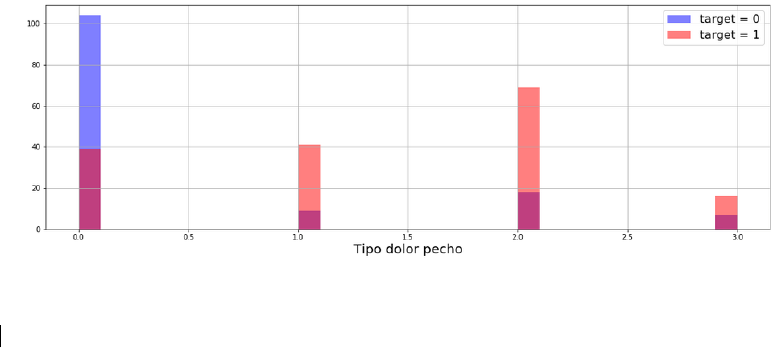239
http://doi.org/10.17993/3ctecno.2020.specialissue5.233-247
3C Tecnología. Glosas de innovación aplicadas a la pyme. ISSN: 2254 – 4143 Edición Especial Special Issue Abril 2020
prole. No single learning algorithm can uniformly outperform other algorithms in all data
sets.
Dierent data sets with dierent types of variables and the number of instances determine
the kind of algorithm that will work well (Manzoor & Singla, 2019). There is no single
learning algorithm that exceeds other algorithms based on all data sets according to the
free lunch theorem. The following table presents a comparative analysis of several learning
algorithms.
3. METHODOLOGY
The methodology to determine the best-supervised algorithm applied in the heart disease
dataset will begin with the interpretation of the data, the preprocessing of the data, and the
application of the algorithms to determine the best accuracy.
A. Dataset
The dataset used for this research will be “Heart Disease” which was found in the Kaggle
repository, this database contains 76 attributes, but all published experiments refer to the
use of a subset of 14 of them. In particular, the Cleveland database is the only one that ML
researchers have used to date. The “goal” eld refers to the presence of heart disease in
the patient. It has an integer value of 0 (no presence) to 4. Experiments with the Cleveland
database have concentrated on the simple attempt to distinguish presence (values 1, 2, 3, 4)
from absence (value 0) (Ray, 2018; Sethi et al., 2019; Agarwal & Sagar, 2019).
B. Interpretation of the data
Next, the data extracted is interpreted from the empirically chosen database.
Figure 1. Type of chest pain.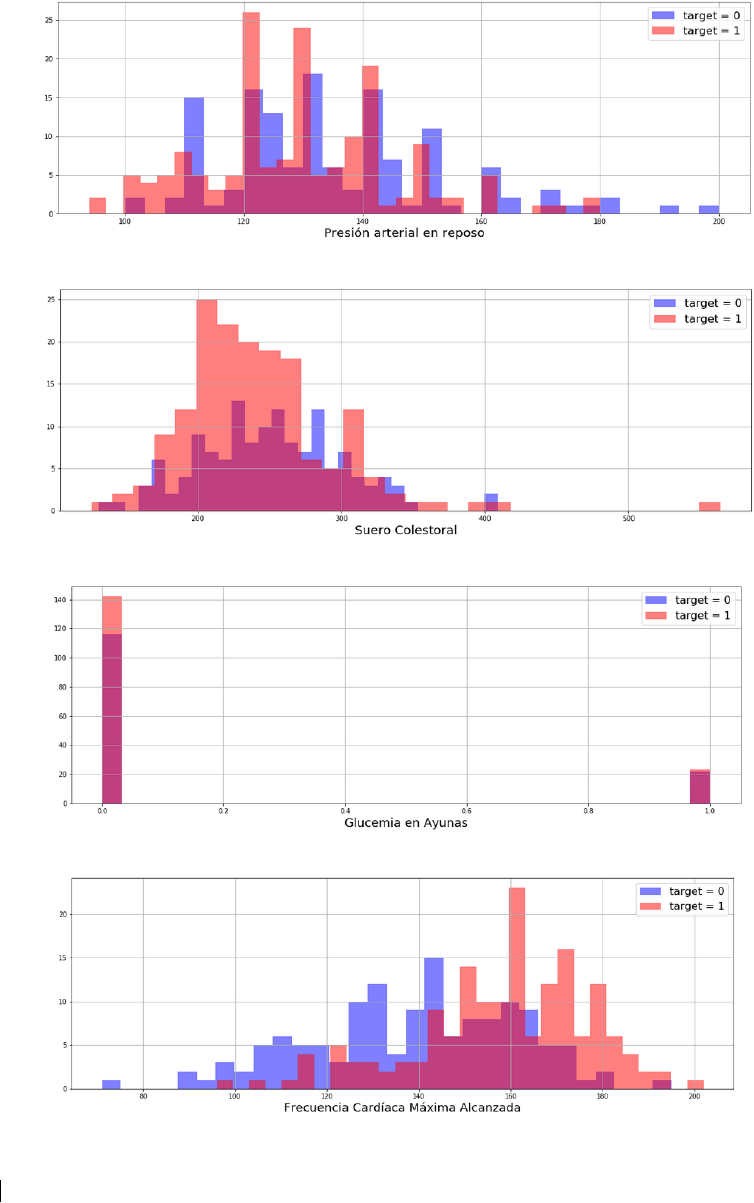240
http://doi.org/10.17993/3ctecno.2020.specialissue5.233-247
3C Tecnología. Glosas de innovación aplicadas a la pyme. ISSN: 2254 – 4143 Edición Especial Special Issue Abril 2020
Figure 2. Resting blood pressure.
Figure 3. Serum cholesterol.
Figure 4. Fasting blood sugar.
Figure 5. Maximum heart rate reached.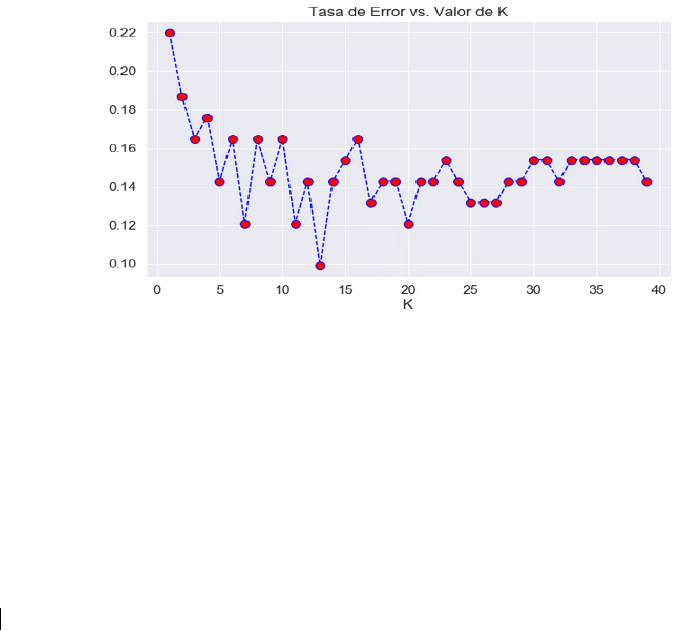241
http://doi.org/10.17993/3ctecno.2020.specialissue5.233-247
3C Tecnología. Glosas de innovación aplicadas a la pyme. ISSN: 2254 – 4143 Edición Especial Special Issue Abril 2020
From the visualization of gures 1,2,3,4, and 5 by category is possible to observe how the
data are expressed, which makes it possible to detect if there is a probability of heart disease.
C. Application of algorithms
After understanding the data and interpreting the information to be generated, the following
algorithms will be applied.
1. K Nearest Neighbors (KNN)
Because the KNN algorithm classier predicts the class of a given test observation by
identifying the observations that are closest to it, the scale of the variables is essential.
Any variable that has a large scale will have a much more signicant eect on the distance
between the observations than the variables that are on a small scale, and therefore on the
KNN classier (Sethi et al., 2019; Agarwal & Sagar, 2019; Cao et al., 2019; Manzoor &
Singla, 2019).
After determining the training and test data with the preprocessing processes, let’s use the
elbow method to choose a good value of K.
Figure 6. Error rate vs. K-value.
Here we can see the error rate after applying K = 13, let’s re-enter the model with this data,
and this information is reached.
1. Decision trees: The data is divided into a training set and a test set, then a single
decision tree will be trained, using the sklearn library, to evaluate the created decision
tree.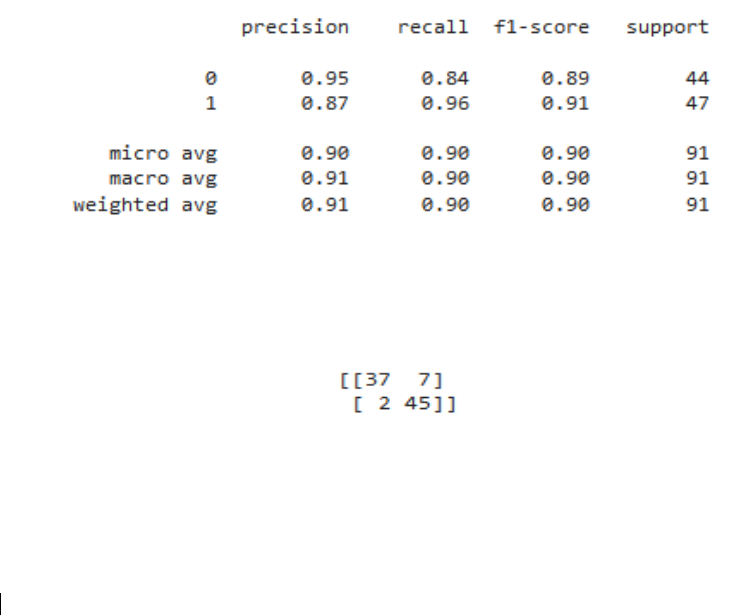242
http://doi.org/10.17993/3ctecno.2020.specialissue5.233-247
3C Tecnología. Glosas de innovación aplicadas a la pyme. ISSN: 2254 – 4143 Edición Especial Special Issue Abril 2020
2. Random Forest: The data is preprocessed, and the training and test variables are
separated to train the model.
3. Neural Network: The sklearn library will be used to preprocess the data to prepare
for training.
4. Support Vector Machines: The data is preprocessed to apply the algorithm, the
training and test variables are separated; we train the model using the sklearn library.
5. RESULTS
After applying the selected supervised learning algorithms to the dataset chosen for
comparison, the following algorithm results are obtained.
A. K Nearest Neighbors (KNN)
To evaluate the model test data was used to nd the confusion matrix, with which we can
calculate the accuracy, precision, recall, and f1-score metrics, the following information is
available:
Table 1. Result of applying the KNN algorithm.
Table 1 shows the average weight as 0.91, and the accuracy formula that is the sum of the
real positives with the true negatives among the total population is applied, an accuracy of
45,614 is reached, and confusion matrix as :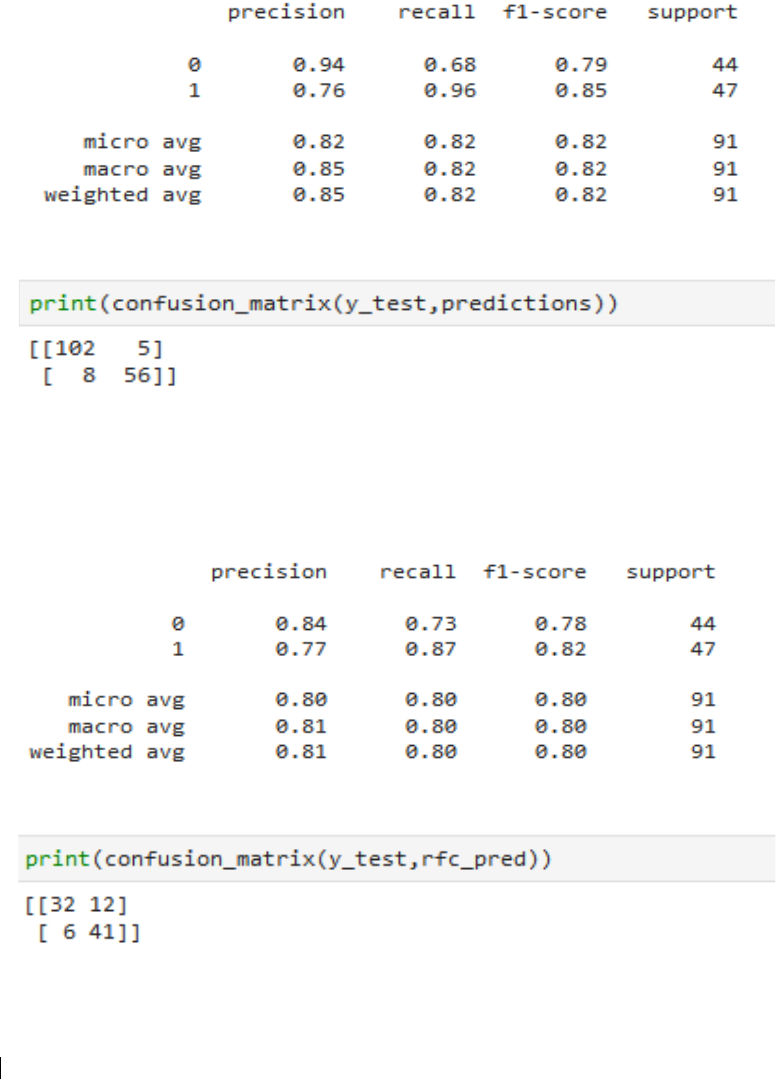243
http://doi.org/10.17993/3ctecno.2020.specialissue5.233-247
3C Tecnología. Glosas de innovación aplicadas a la pyme. ISSN: 2254 – 4143 Edición Especial Special Issue Abril 2020
B. Decision Trees
Applying the decision tree, we get the following results.
Table 2. Result of applying the Decision Trees algorithm.
Table 2 shows the average weight as 0.85, and confusion matrix as:
C. Random Forest
We evaluate the random forest model according to the data already preprocessed and
trained with several estimates of 100.
Table 3. Result of applying the Random Forest algorithm.
It has an average weight of 0.81, and the confusion matrix as: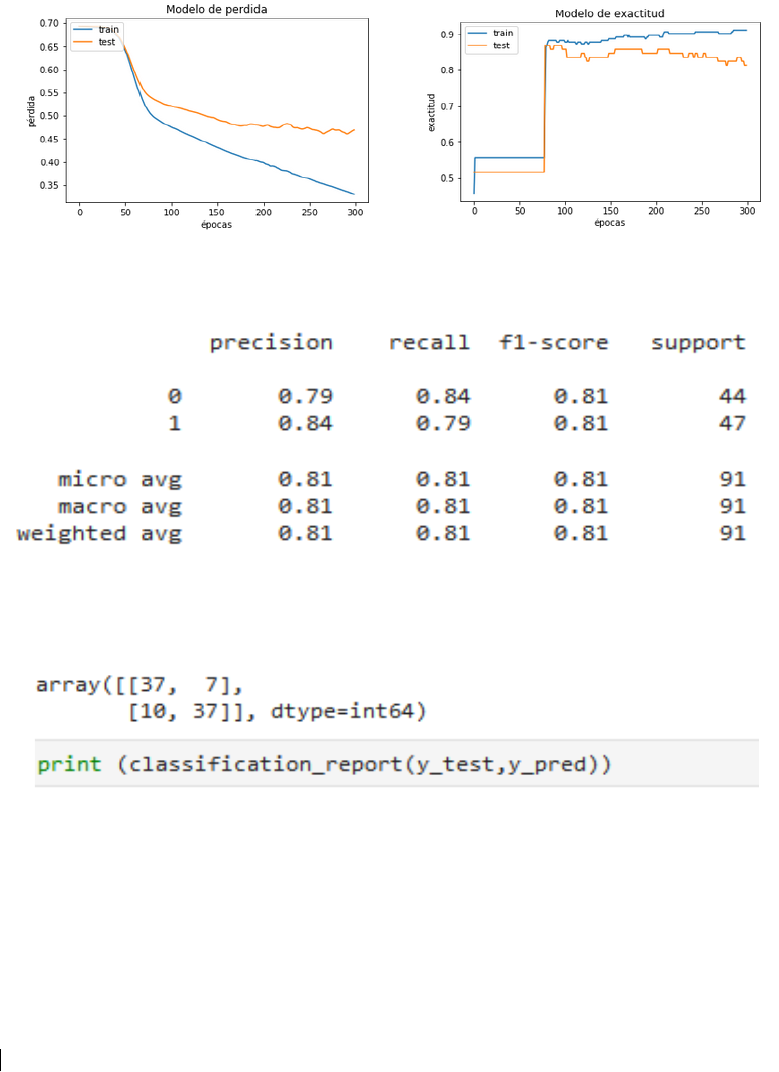244
http://doi.org/10.17993/3ctecno.2020.specialissue5.233-247
3C Tecnología. Glosas de innovación aplicadas a la pyme. ISSN: 2254 – 4143 Edición Especial Special Issue Abril 2020
D. Neural Network
Training and test data are separated, to train the model using Keras dataset, then the model
will be evaluated. Figures 7 and 8 show the models.
Figure 7. Loss model. Figure 8. Accuracy model.
Table 4. Result of applying Neural Network algorithm.
Table 4 shows the weight average accuracy obtained of 0.81.
The following confusion and information matrix are obtained:
E. Support Vector Machines (SVM)
The model will be evaluated according to the preprocessed data, and the following is
obtained, and the report classication and matrix are: